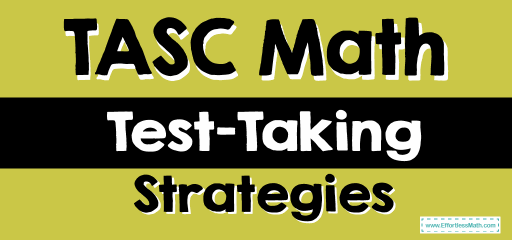# TASC Math Test-Taking StrategiesIf you are preparing for the TASC Math Test, following TASC Math test-taking strategies will help you perform better on the test and increase your test score.

## 1- Use This Approach to Answer Every TASC Math Problem

• Study your problem and identify vital information and relevant keywords
• Convert keywords into mathematical operations to help solve the problem.
• Review the answer choices and find out the difference between them.
• Find patterns in the math problem.
• Create drawings or diagrams where necessary.
• Use straightforward math methods, backsolving, or plug-in numbers to find the correct answers.
• Always double-check after solving a problem.

## 2- Backsolving

Most of the questions you find on the TASC Math test are multiple-choice questions. This type of question is better for many test-takers because the correct answer is provided in the problem.

In the multiple-choice questions, you need to pick the right answer from four or five answer choices. One of the best ways to solve this kind of TASC Math problem is to use the backsolving method.

First, try to answer the question using straightforward math, which entails reading through a math problem, calculating the answer, and looking for the option it corresponds with.

If this method doesn’t work, try to backsolve the problem. To backsolve means to place your answer in contrast with the question you are solving and find out which is the most relevant. Most times, your answers come in descending or ascending order. Choose options B or C, and if they aren’t correct, solve downwards or upwards from there.

## 3- Ballpark

When test-takers face lots of figures and calculations, it can get overwhelming, and this situation can lead to confusion and mistakes. If you get your answer after a rigorous process and then miss the position of a point, all your efforts will waste. Ballparking can help you in such situations.

When you have an idea of the possible answer (even if it is a rough approximation), then you can eliminate several choices that lie outside your approximation.

Seek the choices that don’t fall into the range of your ballpark and set them aside as wrong answers. This method makes it easy to eliminate the wrong answers to a math problem, and then work with the other options to find the correct answer.

## 4- Make Educated Guesses

When faced with problems that you know to a certain extent but cannot solve with a math formula or system, educated guessing is most relevant.

The first step is to attempt filtering out many choices before working on the final answer.

If you have no clue about the answer, attempt to remove choices that might be wrong. However, if you don’t have enough idea about the problem, there is no need to eliminate the options. Just make an educated guess.

Before making your guess, attempt to read through the problem and see if the solution is related to any math question that you have solved before. If it isn’t, make an educated guess and move to the next question.

It is crucial to never leave any questions unanswered. If you cannot solve a problem, answer it using the guess method. It won’t hurt to attempt an answer, but it will be great if you get it right.

## 5- Pluging in Numbers

You can apply the strategy of plugging in numbers to a range of TASC math problems. The method helps you to understand difficult questions by simplifying them.

When you use the ‘plugging in numbers’ method, you have to replace the unknown variables in every math problem with a selected value.

Here are tips to guide you in selecting a number/value.

• Choose a basic number. However, avoid using 0 and 1 because the numbers are too simple. Number 2 works just fine.
• If you need to choose more than one number, choose different numbers.
• Don’t choose a number that is present in the math problem.
• Most of the time, choosing a number only helps you to eliminate some answer choices. So, it is crucial not to select the first answer you get.
• When handling math problems containing percentages, use 100 as the selected value.
• If the problem contains fractions, your answer will likely be the lowest common denominator (LCD) or a multiple.
• When it seems like several answers are correct, try again with another value, and aim to check out choices that you haven’t eliminated already.

## More from Effortless Math for TASC Test …

With so many TASC prep books to choose from on the market, finding the right one is a challenging job. Check out our Top 10 TASC Math Prep Books to find the right prep book for your TASC Math preparation.

### Have you ever wondered what formulas do you need to know for the TASC Math before the test day?

Perhaps you might have heard a lot of things about GED, TASC, and HiSET exams, and you wanted to know Which Test is better for You; GED, TASC, or HiSET? Great! Join the Effortless Math team to review these high school equivalence tests.

## Have any questions about the TASC Test?

### What people say about "TASC Math Test-Taking Strategies - Effortless Math: We Help Students Learn to LOVE Mathematics"?

No one replied yet.

X
52% OFF

Limited time only!

Save Over 52%

SAVE \$40

It was \$76.99 now it is \$36.99